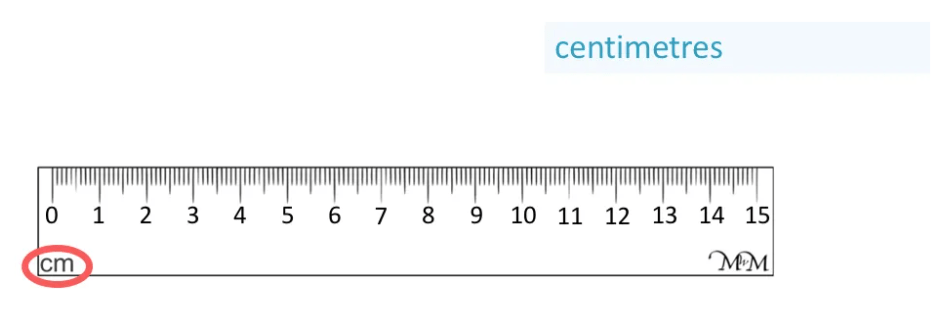# Metric Units of Volume

Metric Units of Volume• The most common metric units of volume are millilitres, litres and kilolitres.
• A millilitre is the size of a droplet of water.
• There are 1000 millilitres in a litre.
• A litre is the size of a large bottle of water.
• There are 1000 litres in a kilolitre.
• A kilolitre is the same size as a cube that has sides 1 metre long.
• Millilitres are written as mL, litres are written as L and kilolitres are written as kL.
The most common metric units of volume are millilitres (mL), litres (L) and kilolitres (kL).

There are 1000 mL in 1 L and 1000 L in 1 kL.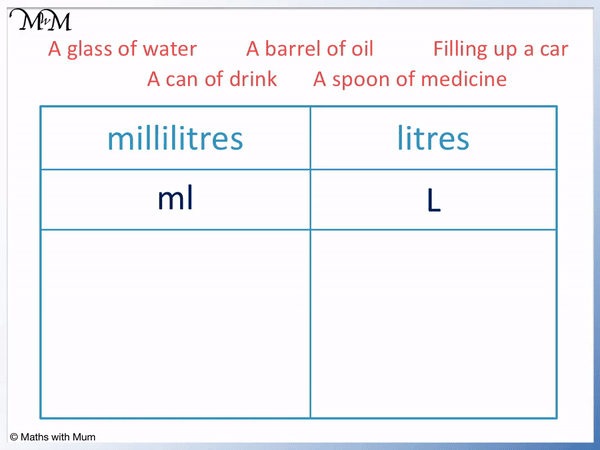• In general, millilitres are used to measure any volume that is smaller than 1 litre and litres are used to measure any volume that is 1 litre or more.
• A litre is about the size of a large bottle, so volumes larger than a large bottle are measured in litres and volumes less than a large bottle are measured in millilitres.
• A glass of water, a can of drink and a spoon of medicine are all smaller than a large bottle and so, they are measured in mL.
• A barrel of oil is larger than a large bottle and a car engine needs more petrol than a large bottle contains. Therefore both of these volumes are measured in litres.
• Kilolitres are used less frequently than litres and are chosen to measure very large amounts.
• Some examples of things measured in kilolitres are the amount of water in swimming pools and the amount of water used by a house in each quarterly bill.# Metric Units of Volume

## What are the Units of Volume?

The most commonly used units of volume from smallest to largest are:

Unit of Volume Abbreviation Equivalent Size Example
1 millilitre 1 ml = 1 cm3 A raindrop
1 centilitre 1 cl = 10 cm3 2 teaspoons
1 litre 1 L = 1000 cm3 A bottle of dish washing liquid
1 kilolitre 1 kL = 1 m3 8 full bathtubs of water

Millitres are used to measure small quantities of liquid, typically less than 1 litre. Examples of items measured in millilitres are medicine containers, drink cartons and shampoo.

Centilitres are used to measure small quantities of liquid such as wine in a glass and engine capacity. Centilitres are used less frequently to measure capacity than millilitres or litres are.

Litres are used to measure most quantities of liquid that are greater than a large drink bottle. Examples of items measured in litres are bottles of milk, petrol in a car and buckets of water.

Kilolitres are used to measure very large quantities of liquid such as oil in a storage tank, water in a fire truck and the amount of water used for the water bill of a household.

## How Much is 1 Millilitre?

1 millilitre is the size of a droplet of rain. 5 millilitres fit on a level teaspoon and 250 millilitres is the same amount in a small carton of juice. 1000 millilitres make one litre.

Millilitres is written as milliliters in the USA. Millilitres is written as mL or ml for short.

1 mL is the amount of water in a single droplet.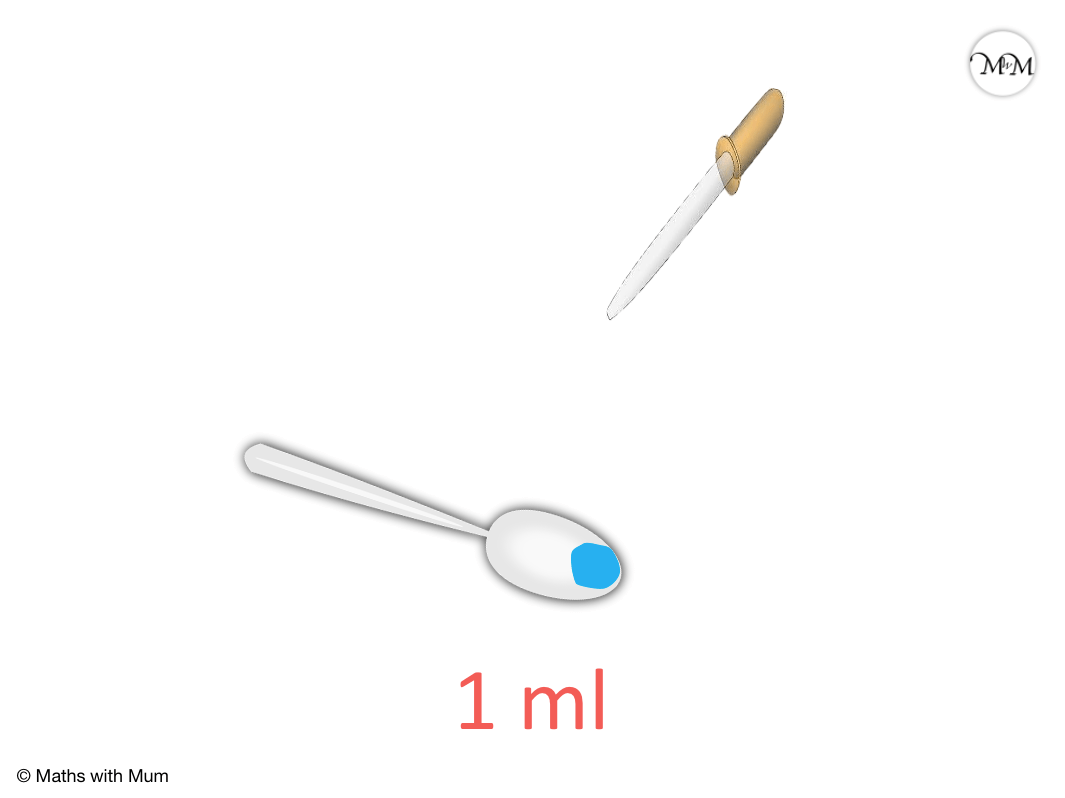Millilitres are often used to measure liquid in measuring flasks. Below are three different flasks showing 30 mL, 50 mL and 100 mL.

30 mL is about the size of one mouthful. 100 mL is the size of a small tube of toothpaste.A carton of juice contains 250 mL. A can of soft drink contains 375 mL.A typical bottle of drink that is for one person to consume contains 500 mL.## How Much is 1 Litre?

1 litre is equivalent to 1000 millilitres and it is the amount of liquid in a large water bottle. 2 litres is enough to fill a kettle to the brim and there are about 100 litres in a bathtub.

1000 millilitres make 1 litre.

1 litre is approximately the size of a large drink bottle that can be held in one hand. It is twice as much as the typical drink bottle sizes sold in vending machines.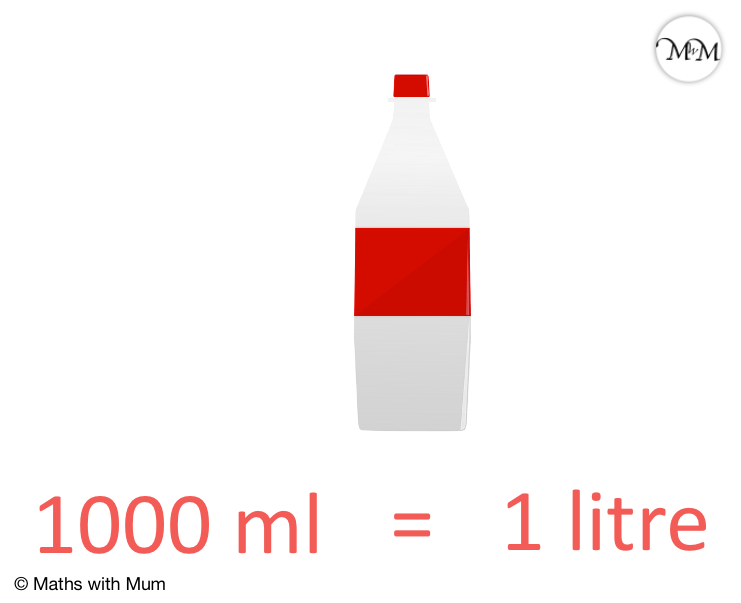The largest bottles of drinks that can be bought in supermarkets contain 2 litres. 2L is the amount found in the family size drink bottles.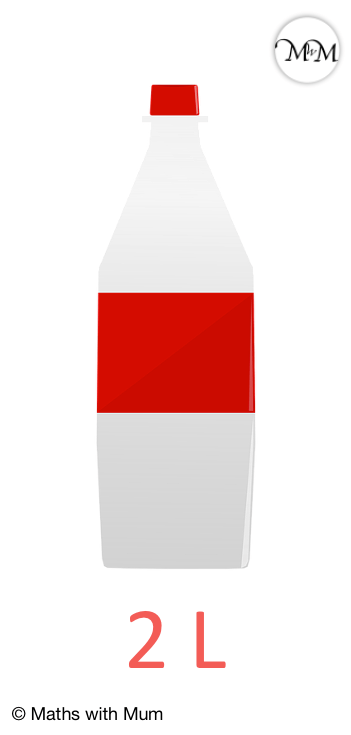Litres are used to measure most quantities of capacity larger than 1 litre.

For example, filling a car requires about 50 litres.A full bathtub contains approximately 150 litres of water.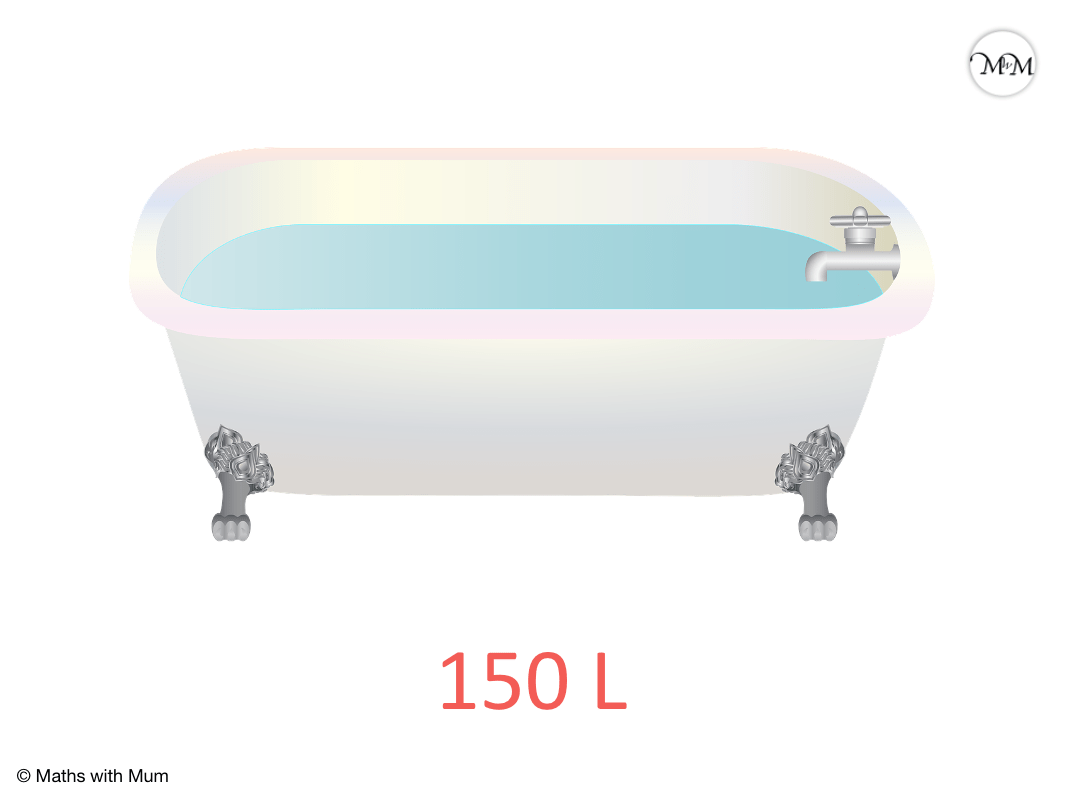A wine barrel is an example of something measured in litres. There are approximately 200 litres of wine in a wine barrel.## How Much is 1 Kilolitre?

1 kilolitre is as large as 1000 litres. 1 kilolitre is as much water as 8 full bathtubs. There are 2,500 kilolitres of water in an olympic sized swimming pool.

Typical water bills measure the amount of water used by a household each time period in kilolitres. The price of water is given in cost per kilolitre.

## How to Convert Units of Volume

To convert between metric units of volume, use the following rules:

Unit of Volume Conversion
1 kL = 1000 L
1 L = 1000 mLKilo means 1000. Therefore 1 kilolitre contains 1000 litres. To convert from kilolitres to litres, simply multiply the amount by 1000. For example, 3 kL = 3000 L.

To convert from L to kL, divide the value by 1000. For example, 257 L = 0.257 kL.

Milli means one-thousandth. Therefore 1 litre contains 1000 millilitres. To convert from litres to millilitres, simply multiply the amount by 1000. For example, 5 L = 5000 mL.

To convert from mL to L, divide the value by 1000. For example, 375 mL = 0.375 L.

## Choosing Appropriate Units of Volume

The most common units of volume are millilitres and litres. Millilitres are chosen to measure volumes less than 1 litre (about the size of a large bottle). Litres are chosen to measure volumes equal to 1 litre or greater.As a general rule, if the amount being measured is less than the size of a large bottle of water, it should be measured in mL. If the amount is larger than a large bottle, it should be measured in L.

For example, a glass of water, a can of drink and a dose of medicine are all measured in millilitres because they are all less than 1 litre.

It is better to say that a can is 375 mL instead of 0.375 L. This is because it is easier to visualise how much a whole number is compared to a decimal number. Each can contains 375 millilitre drops.Filling up a car is measured in litres because it is a relatively large amount of volume. It is better to find whole number values that are not too large (larger than 1000).

It is better to say that the car contains 50 L instead of 50, 000 mL. We are also filling the car quickly, so we can measure each litre as it fills the car. It would be too difficult to fill the car millilitre by millilitre as it comes out too quickly.

Similarly, a barrel of oil would be measured in litres as it is larger than a large bottle. It is better to say that the barrel contains 200 L instead of 200, 000 mL.Now try our lesson on Measuring Centimetres Using a Ruler where we learn how to measure objects correctly using a ruler.